# Basic Operations On Stock Data Using Python

This article illustrates basic operations that can be performed on stock data using Python to analyze and build algorithmic trading strategies. We run through some basic operations that can be performed on a stock data using Python and we start by reading the stock data from a CSV file.

Python has emerged as the fastest-growing programming language and this has stemmed from multiple factors like ease to learn, readability, conciseness, strong developer community, application across domains etc.Python has found wide acceptance in trading too and this has led to Python-based analytics platforms, Python APIs, and trading strategies being built using Python.

Given the growing popularity and ease to learn, the Executive Programme in Algorithmic Trading (EPAT) offers a dedicated module which covers Quantitative Trading Strategies taught using Python.

The objective of this post is to illustrate how easy it is to learn Python and apply it to formulate and analyze trading strategies. If you are new to programming this blog might just help you overcome your fear of programming. Also, don’t forget to check out some nice links provided at the end of this blog to learn some exciting trading strategies which have been posted on our blog.

Let us run through some basic operations that can be performed on stock data using Python. We start by reading the stock data from a CSV file. The CSV file contains the Open-High-Low-Close (OHLC) and Volume numbers for the stock.

import pandas as pd
# Load data from csv filedata = pd.read_csv('Basic Operations on Stock Data using Python_UBL.csv')print(data.head())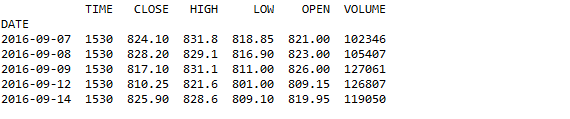The 'TIME' column seen here specifies the closing time of the day’s trading session. To delete the column we can simply use the 'del' command.

# Deleting the "TIME" column
del data['TIME']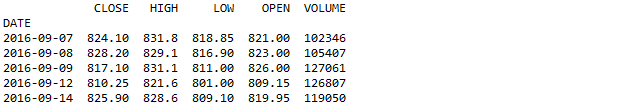Now, let us use the type function to check whether the object is a pandas datetime index.

type(data.index)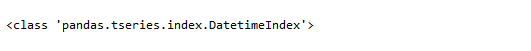I would like to know the number of trading days (the number of rows) in the given data set. It can be done using the count method.

# Number of rows in the data set
print(data['CLOSE'].count())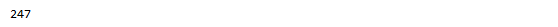What if I want to know the maximum close price that was reached in the given period? This is made possible by using the max method.

max_price = data['CLOSE'].max()
print(max_price)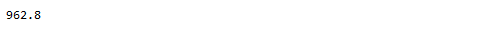Is it also possible to know the date on which this maximum price was reached? To find the respective date we apply the index property as shown below.

data.CLOSE[data.CLOSE == max_price].index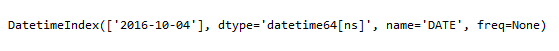Let us compute the daily percentage change in closing price. We add a new column of 'Percentage_Change' to our existing data set. In the next line of code, we have filtered the per cent change column for all the values greater than 1.0. The result has been presented below.

# Compute the percentage change
data['Percent_Change'] = data['CLOSE'].pct_change()*100
# Filter the percent change column for all values greater than 1.0
dt = (data[data.Percent_Change > 1.0])
print(dt.head())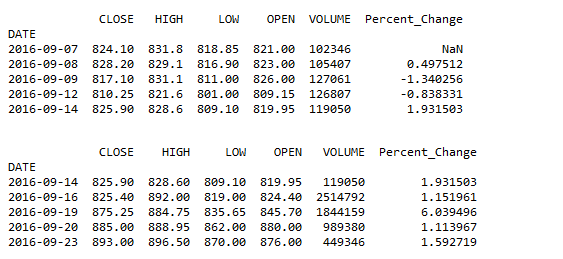Finally, let us add a couple of indicators. We compute the 20-day simple moving average and the 5-day average volume. We can add more indicators to our data frame and then analyze the stock trend to see whether it is bullish or bearish. You can learn more on how to create various technical indicators in Python here.

# Closing near the 20-day SMA
ndays = 20
SMA = pd.Series((data['CLOSE']).rolling(window=ndays).mean(),name = 'SMA')
data = data.join(SMA)
Avg_vol = pd.Series((data['VOLUME']).rolling(window=5).mean(),name = '5day_AvgVol')
data = data.join(Avg_vol)
print(data.tail(7))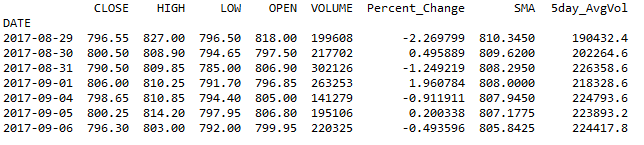In his short post, we covered some simple ways to analyze the data set and build more understanding of the stock data.

Can you think of building a trading strategy using similar basic operations and simple indicators?

Simple trading strategies can be profitable and many successful traders will vouch for that. As mentioned at the start of the blog, here are the links to some trading strategies in Python that can be explored for your own trading needs.